# Corrective Mathematics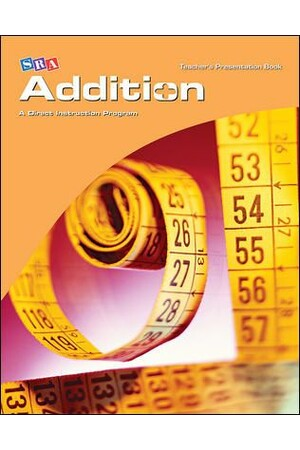### Corrective Mathematics - Addition: Teacher Materials

\$251.95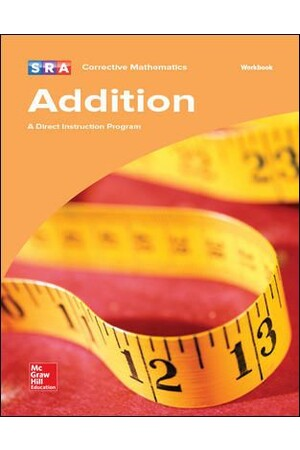### Corrective Mathematics - Addition: Workbook

\$26.95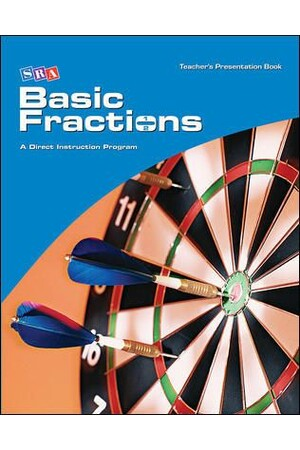### Corrective Mathematics - Basic Fractions: Teacher Materials

\$251.95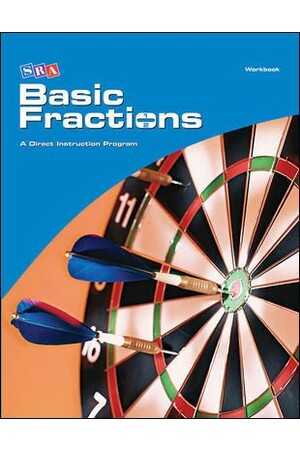### Corrective Mathematics - Basic Fractions: Workbook

\$26.95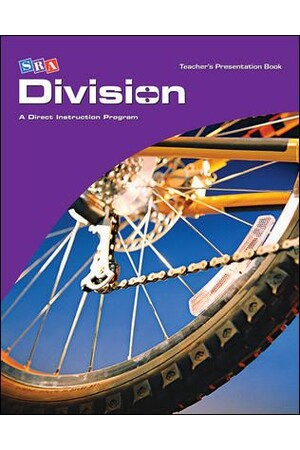### Corrective Mathematics - Division: Teacher Materials

\$251.95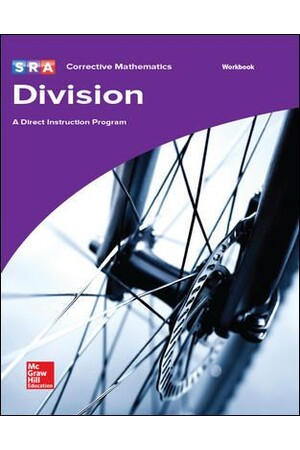### Corrective Mathematics - Division: Workbook

\$26.95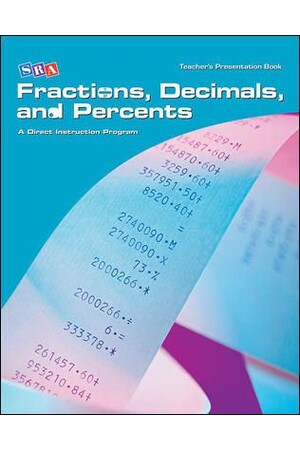### Corrective Mathematics - Fractions, Decimals, and Percents: Teacher Materials

\$251.95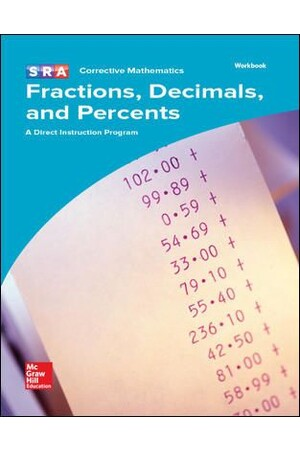### Corrective Mathematics - Fractions, Decimals, and Percents: Workbook

\$26.95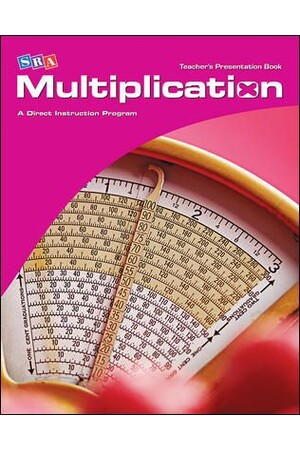### Corrective Mathematics - Multiplication: Teacher Materials

\$251.95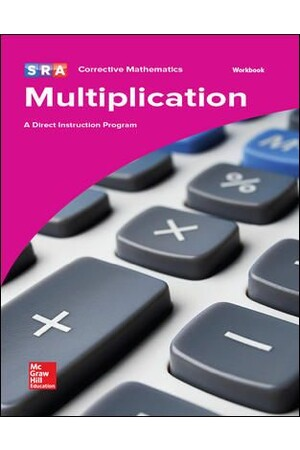### Corrective Mathematics - Multiplication: Workbook

\$26.95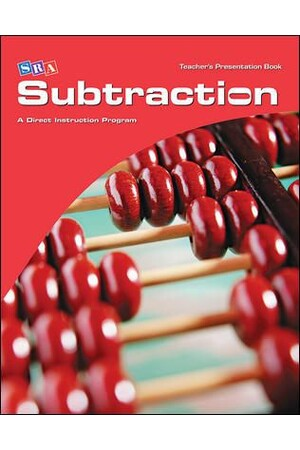### Corrective Mathematics - Subtraction: Teacher Materials

\$251.95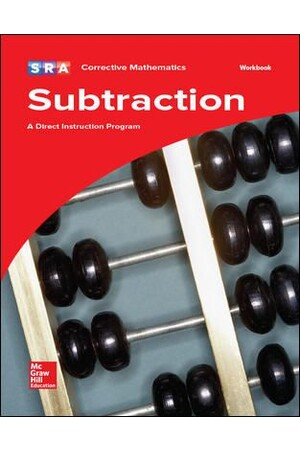\$26.95